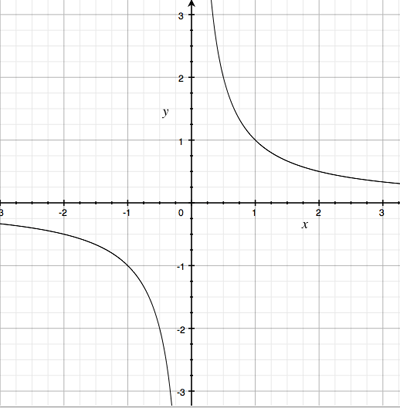SEARCH HOMEMath Central Quandaries & QueriesQuestion from Lucy, a student: What is the range of the function: h(x)=1/x (and can you please explain why)?Hi Lucy,

Sketch the graph.From the graph it looks like the range of h is all real numbers except zero. Can you prove it?

Suppose that $a$ is a real number and $a \neq 0.$ Can you find a real number $x$ so that $h(x) = a?$

Can you find a real number $x$ so that $h(x) = 0?$ Why not?

PennyMath Central is supported by the University of Regina and The Pacific Institute for the Mathematical Sciences.